# Contents

Package: similaRpeak
Authors: Astrid Deschenes [cre, aut], Elsa Bernatchez [aut], Charles Joly Beauparlant [aut], Fabien Claude Lamaze [aut], Rawane Samb [aut], Pascal Belleau [aut], Arnaud Droit [aut]
Version: 1.6.0
Compiled date: 2016-10-17

# 1 Metrics which estimate similarity between two ChIP-Seq profiles

Astrid Deschenes, Elsa Bernatchez, Charles Joly Beauparlant, Fabien Claude Lamaze, Rawane Samb, Pascal Belleau and Arnaud Droit.

This package and the underlying similaRpeak code are distributed under the Artistic license 2.0. You are free to use and redistribute this software.

## 1.1 Introduction

The similaRpeak package calculates metrics to estimate the level of similarity between two ChIP-Seq profiles.

The metrics are:

• RATIO_AREA: The ratio between the profile areas. The first profile is always divided by the second profile. NA is returned if minimal area threshold is not respected for at least one of the profiles.

• DIFF_POS_MAX: The difference between the maximal peaks positions. The difference is always the first profile value minus the second profile value. NA is returned if minimal peak value is not respected. A profile can have more than one position with the maximum value. In that case, the median position is used. A threshold argument can be set to consider all positions within a certain range of the maximum value. A threshold argument can also be set to ensure that the distance between two maximum values is not too wide. When this distance is not respected, it is assumed that more than one peak is present in the profile and NA is returned.

• RATIO_MAX_MAX: The ratio between the peaks values in each profile. The first profile is always divided by the second profile. NA if minimal peak value threshold is not respected for at least one of the profiles.

• RATIO_INTERSECT: The ratio between the intersection area and the total area of the two profiles. NA if minimal area threshold is not respected for the intersection area.

• RATIO_NORMALIZED_INTERSECT: The ratio between the intersection area and the total area of two normalized profiles. The profiles are normalized by dividing them by their average value. NA if minimal area threshold is not respected for the intersection area.

• SPEARMAN_CORRELATION: The Spearman’s rho statistic between profiles.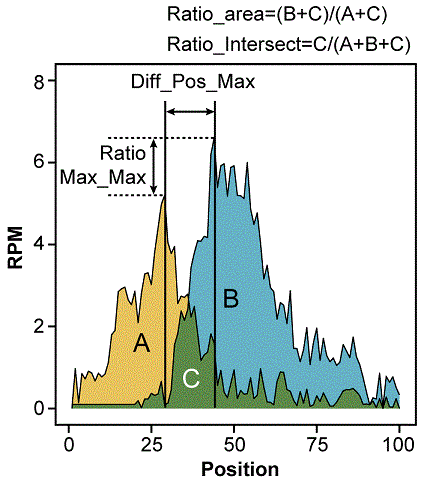suppressMessages(library(similaRpeak))

## 1.3 Inputs

### 1.3.1 ChIP-Seq profiles vectors

ChIP-seq combines chromatin immunoprecipitation (ChIP) with massively parallel DNA sequencing to identify the binding sites of DNA-associated proteins. For a specific region, the read count of aligned sequences at each position of the region is used to generate the ChIP-Seq profile for the region.

To estimate the level of similarity between two ChIP-Seq profiles for a specific region, two vector containing the profiles values, as reported in reads per million (RPM) for each position of the selected region, are needed. Both vector should have the same length and should not contain any negative value.

Aligned sequences are usually stored in BAM files. As example, a slimmed BAM file (align1.bam) is selected as well as a specific region (chr1:172081530-172083529). Using BAM file and region information, represented by as a GRanges object, the coverage for the specified region is extracted using specialized Bioconductor packages.

suppressMessages(library(GenomicAlignments))
suppressMessages(library(rtracklayer))
suppressMessages(library(Rsamtools))

bamFile01 <- system.file("extdata/align1.bam", package = "similaRpeak")

region <- GRanges(Rle(c("chr1")), IRanges(start = 172081530, end = 172083529),
strand= Rle(strand(c("*"))))

param <- ScanBamParam(which = region)

alignments01 <- readGAlignments(bamFile01, param = param)

coveragesRegion01 <- coverage(alignments01)[region]
coveragesRegion01
## RleList of length 1
## $chr1 ## integer-Rle of length 2000 with 92 runs ## Lengths: 297 30 2 4 14 18 19 36 ... 21 15 35 36 65 36 307 ## Values : 0 1 2 3 2 1 0 1 ... 2 1 0 1 0 2 0 The coverages01 can easily be transformed to a vector of numerical value to obtain the raw ChIP-Seq profile for the selected region. coveragesRegion01 <- as.numeric(coveragesRegion01$chr1)
length(coveragesRegion01)
##  2000
summary(coveragesRegion01)
##    Min. 1st Qu.  Median    Mean 3rd Qu.    Max.
##   0.000   0.000   0.000   0.907   1.000   6.000

To convert the raw coverage to reads per million (RPM), the total number of reads present in the BAM file is needed to assign a weight at the coverage function.

param <- ScanBamParam(flag = scanBamFlag(isUnmappedQuery=FALSE))
count01 <- countBam(bamFile01, param = param)records coveragesRPMRegion01 <- coverage(alignments01, weight=1000000/count01)[region] coveragesRPMRegion01 <- as.numeric(coveragesRPMRegion01chr1)
length(coveragesRPMRegion01)
##  2000
summary(coveragesRPMRegion01)
##    Min. 1st Qu.  Median    Mean 3rd Qu.    Max.
##     0.0     0.0     0.0   195.7   215.7  1294.0

The read per millions values are quite low for the coveragesRPMRegion01 because the original BAM file has been reduced in size to simplify the example.

Other examples are available on the worflows section of the Bioconductor website.

Finally, the metagene package , available on Bioconductor, can also be used to generate ChIP-Seq profiles. An example is available on metagene wiki.

## 1.4 Metrics

### 1.4.1 Metric definition

Mathematically, a metric is considered as a function that quantifies the similarity between two objects.The function must return zero when the two objects are perfectly similar (identity of indiscernibles) and a non-negative value when are dissimilar.

The metrics present in the similaRpeak package do not strictly respect this standard but can all be translated to pseudometrics. A pseudometric is a function d which satisfies the axioms for a metric, except that instead of the identity of indiscernibles axiom, the metric must only return zero when it compare an object to itself.

By using the absolute value of the DIFF_POS_MAX metric, the definition of a pseudometric is formally respected. However, the respective position of the maximum peak of profiles is lost.

$|DIFF\_POS\_MAX|$

By using the absolute value of the logarithm of the RATIO_AREA, RATIO_MAX_MAX, RATIO_INTERSECT and RATIO_NORMALIZED_INTERSECT metrics, the definition of a pseudometric is formally respected.

$|\log(RATIO)|$

### 1.4.2 Metrics demonstration

To ease comparison, the same ChIP-Seq profiles are used in each metric description section. Those are ChIP-Seq profiles of two histone post-transcriptional modifications linked to highly active enhancers H3K27ac (DCC accession: ENCFF000ASG) and H3K4me1 (DCC accession: ENCFF000ARY) from the Encyclopedia of DNA Elements (ENCODE) data (Dunham I et al. 2012).

Here is how to load the demoProfiles data used in following sections. The ChIP-Seq profiles of the enhancers H3K27ac and H3K4me1 for 4 specifics regions are in reads per million (RPM).

data(demoProfiles)
str(demoProfiles)
## List of 4
##  $chr2.70360770.70361098 :List of 2 ## ..$ H3K27ac: num [1:329] 13 12 12 11 11 11 9 9 7 7 ...
##   ..$H3K4me1: num [1:329] 6 7 7 7 7 8 8 8 8 9 ... ##$ chr3.73159773.73160145 :List of 2
##   ..$H3K27ac: num [1:373] 1 0 0 0 0 0 0 0 0 0 ... ## ..$ H3K4me1: num [1:373] 0 0 0 0 0 0 0 0 0 0 ...
##  $chr8.43092918.43093442 :List of 2 ## ..$ H3K27ac: num [1:525] 82 82 81 81 80 80 79 78 78 76 ...
##   ..$H3K4me1: num [1:525] 1404 1398 1392 1383 1367 ... ##$ chr19.27739373.27739767:List of 2
##   ..$H3K27ac: num [1:395] 4 3 3 3 2 2 0 0 0 0 ... ## ..$ H3K4me1: num [1:395] 17 15 13 13 11 6 3 0 0 0 ...

#### 1.4.2.1 RATIO_AREA Metric

The RATIO_AREA metric is the ratio between the profile areas. The first profile (profile1 parameter) is always divided by the second profile (profile2 parameter). NA is returned if minimal area threshold (ratioAreaThreshold parameter) is not respected for at least one of the profiles.

The RATIO_AREA metric can be useful to detect regions with similar coverage even if the profiles are different.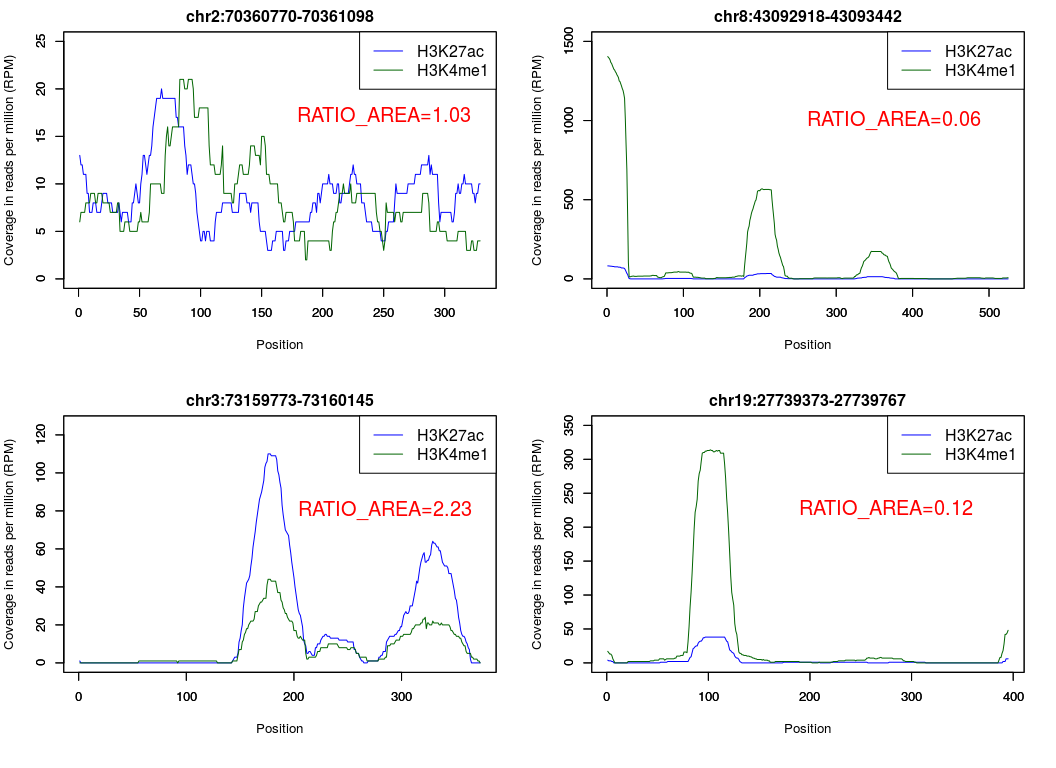metrics <- similarity(demoProfiles$chr2.70360770.70361098$H3K27ac,
demoProfiles$chr2.70360770.70361098$H3K4me1)
metrics$metric$RATIO_AREA
##  1.025942

metrics <- similarity(demoProfiles$chr8.43092918.43093442$H3K27ac,
demoProfiles$chr8.43092918.43093442$H3K4me1)
metrics$metric$RATIO_AREA
##  0.05928535

metrics <- similarity(demoProfiles$chr3.73159773.73160145$H3K27ac,
demoProfiles$chr3.73159773.73160145$H3K4me1)
metrics$metric$RATIO_AREA
##  2.228563

metrics <- similarity(demoProfiles$chr19.27739373.27739767$H3K27ac,
demoProfiles$chr19.27739373.27739767$H3K4me1)
metrics$metric$RATIO_AREA
##  0.1245884

#### 1.4.2.2 DIFF_POS_MAX Metric

The DIFF_POS_MAX metric is the difference between the maximal peaks positions. The difference is always the first profile value (profile1 parameter) minus the second profile value (profile2 parameter). NA is returned if minimal peak value is not respected. A profile can have more than one position with the maximum value. In that case, the median position is used. A threshold (diffPosMaxTolerance parameter) can be set to consider all positions within a certain range of the maximum value. A threshold (diffPosMaxThresholdMaxDiff parameter) can also be set to ensure that the distance between two maximum values is not too wide. When this distance is not respected, it is assumed that more than one peak is present in the profile and NA is returned. Finally, a threshold (diffPosMaxThresholdMinValue parameter) can be set to ensure that the maximum value is egal or superior to a minimal value. When this minimum value is not respected, it is assumed that no peak is present in the profile and NA is returned.

The DIFF_POS_MAX metric can be useful to detect regions with shifted peaks.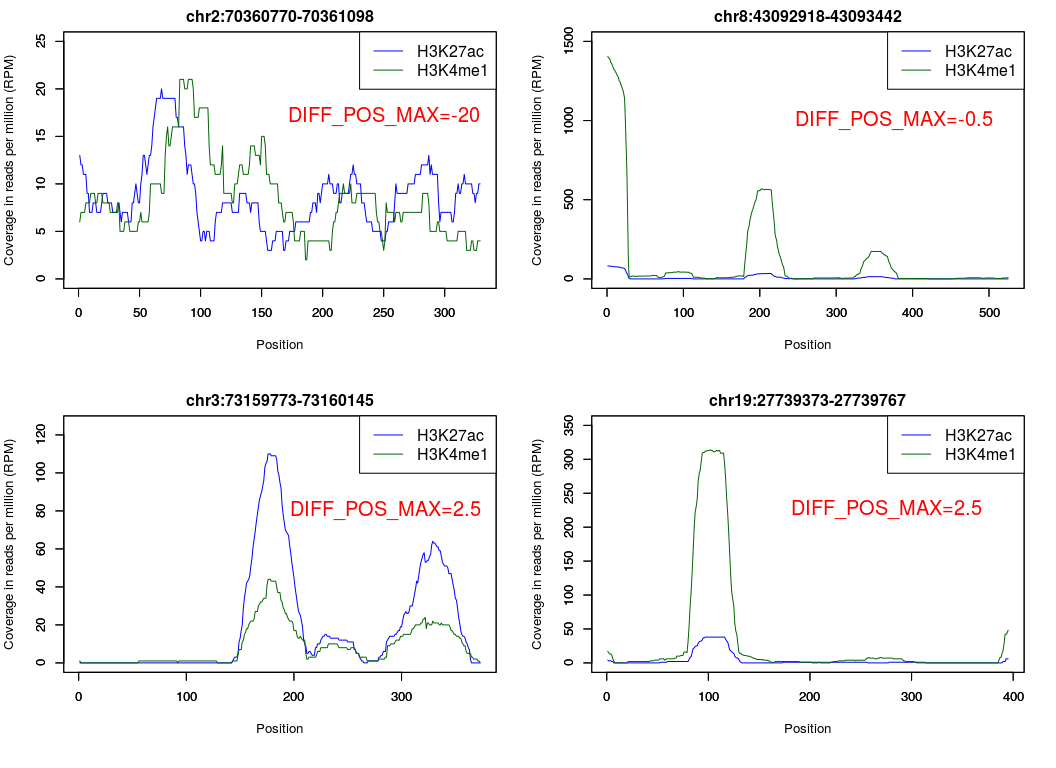metrics <- similarity(demoProfiles$chr2.70360770.70361098$H3K27ac,
demoProfiles$chr2.70360770.70361098$H3K4me1)
metrics$metric$DIFF_POS_MAX
##  -20

metrics <- similarity(demoProfiles$chr8.43092918.43093442$H3K27ac,
demoProfiles$chr8.43092918.43093442$H3K4me1)
metrics$metric$DIFF_POS_MAX
##  -0.5

metrics <- similarity(demoProfiles$chr3.73159773.73160145$H3K27ac,
demoProfiles$chr3.73159773.73160145$H3K4me1)
metrics$metric$DIFF_POS_MAX
##  2.5

metrics <- similarity(demoProfiles$chr19.27739373.27739767$H3K27ac,
demoProfiles$chr19.27739373.27739767$H3K4me1)
metrics$metric$DIFF_POS_MAX
##  2.5

#### 1.4.2.3 RATIO_MAX_MAX Metric

The RATIO_MAX_MAX metric is the ratio between the peaks values in each profile. The first profile (profile1 parameter) is always divided by the second profile (profile2 parameter). NA if minimal peak value threshold (ratioMaxMaxThreshold parameter) is not respected for at least one of the profiles.

The RATIO_MAX_MAX metric can be useful to detect regions with peaks with similar (or dissimilar) amplitude.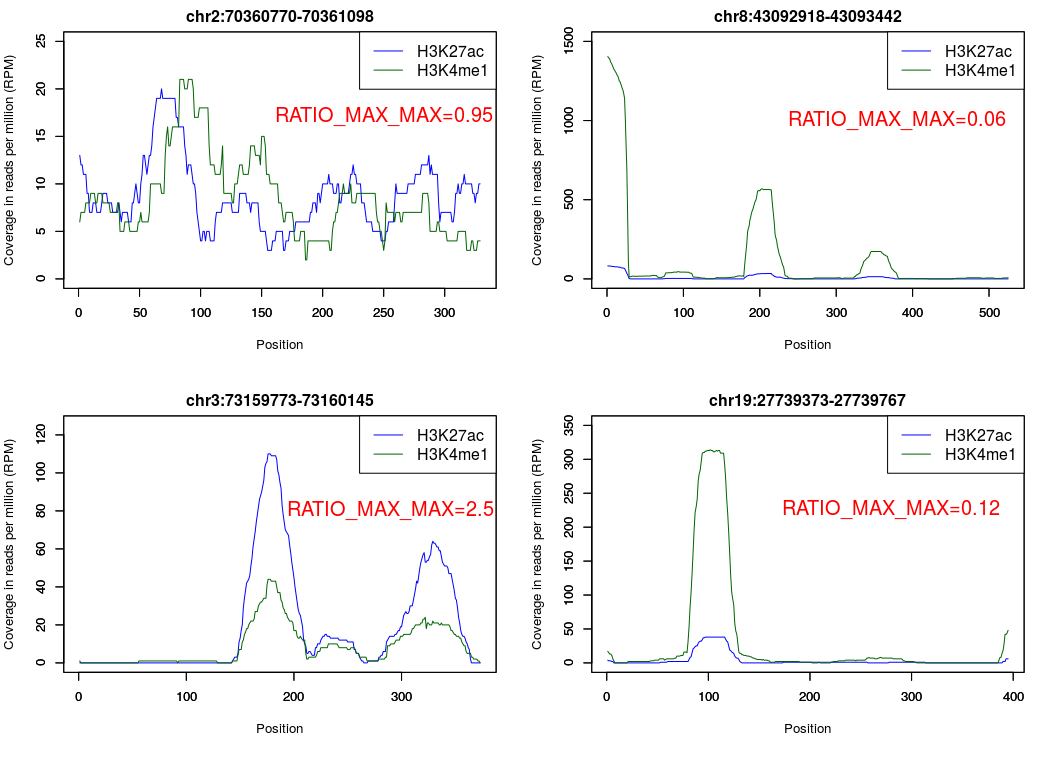metrics <- similarity(demoProfiles$chr2.70360770.70361098$H3K27ac,
demoProfiles$chr2.70360770.70361098$H3K4me1)
metrics$metric$RATIO_MAX_MAX
##  0.952381

metrics <- similarity(demoProfiles$chr8.43092918.43093442$H3K27ac,
demoProfiles$chr8.43092918.43093442$H3K4me1)
metrics$metric$RATIO_MAX_MAX
##  0.05840456

metrics <- similarity(demoProfiles$chr3.73159773.73160145$H3K27ac,
demoProfiles$chr3.73159773.73160145$H3K4me1)
metrics$metric$RATIO_MAX_MAX
##  2.5

metrics <- similarity(demoProfiles$chr19.27739373.27739767$H3K27ac,
demoProfiles$chr19.27739373.27739767$H3K4me1)
metrics$metric$RATIO_MAX_MAX
##  0.1210191

#### 1.4.2.4 RATIO_INTERSECT Metric

The RATIO_INTERSECT metric is the ratio between the intersection area and the total area of the two profiles. NA if minimal area threshold (ratioIntersectThreshold parameter) is not respected for the intersection area.

The RATIO_INTERSECT metric can be useful to detect regions with possibily similar profiles.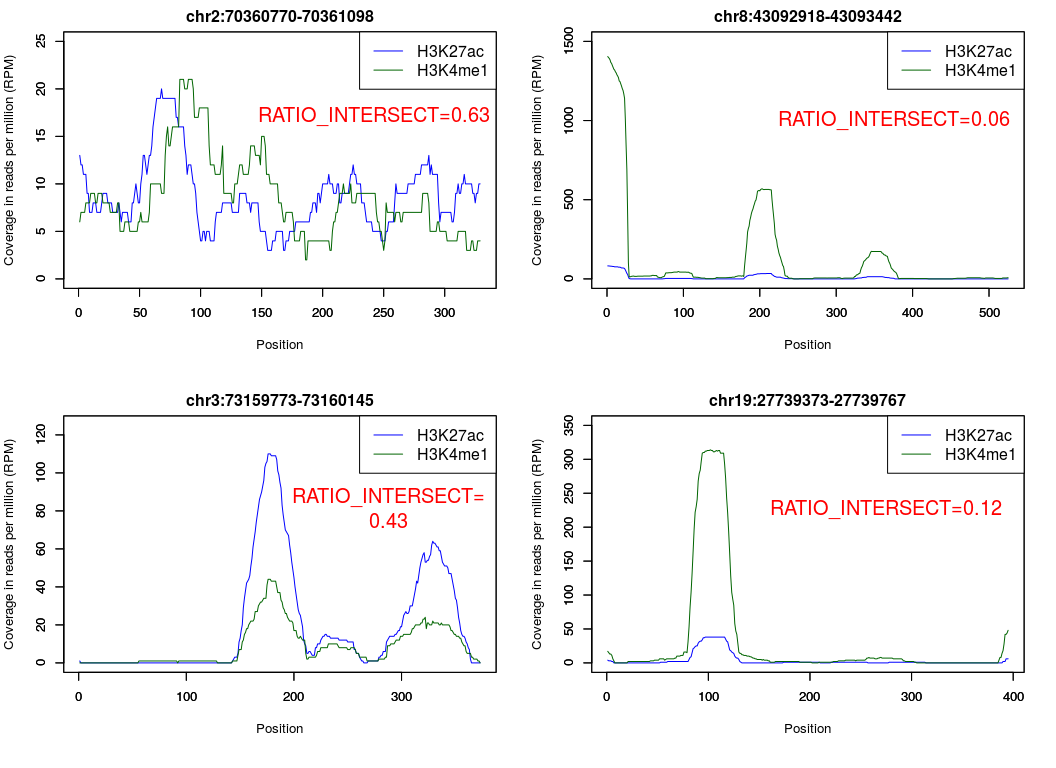metrics <- similarity(demoProfiles$chr2.70360770.70361098$H3K27ac,
demoProfiles$chr2.70360770.70361098$H3K4me1)
metrics$metric$RATIO_INTERSECT
##  0.6307208

metrics <- similarity(demoProfiles$chr8.43092918.43093442$H3K27ac,
demoProfiles$chr8.43092918.43093442$H3K4me1)
metrics$metric$RATIO_INTERSECT
##  0.05926862

metrics <- similarity(demoProfiles$chr3.73159773.73160145$H3K27ac,
demoProfiles$chr3.73159773.73160145$H3K4me1)
metrics$metric$RATIO_INTERSECT
##  0.4276368

metrics <- similarity(demoProfiles$chr19.27739373.27739767$H3K27ac,
demoProfiles$chr19.27739373.27739767$H3K4me1)
metrics$metric$RATIO_INTERSECT
##  0.1231793

#### 1.4.2.5 RATIO_NORMALIZED_INTERSECT Metric

The RATIO_NORMALIZED_INTERSECT metric is the ratio between the intersection area and the total area of the two normalized profiles. The profiles are normalized by divinding them by their average value (total area of the profile divided by profile lenght). NA if minimal area threshold (ratioNormalizedIntersectThreshold parameter) is not respected for the intersection area.

The RATIO_NORMALIZED_INTERSECT metric can be useful to detect regions with possibily similar profiles even if their have different amplitude.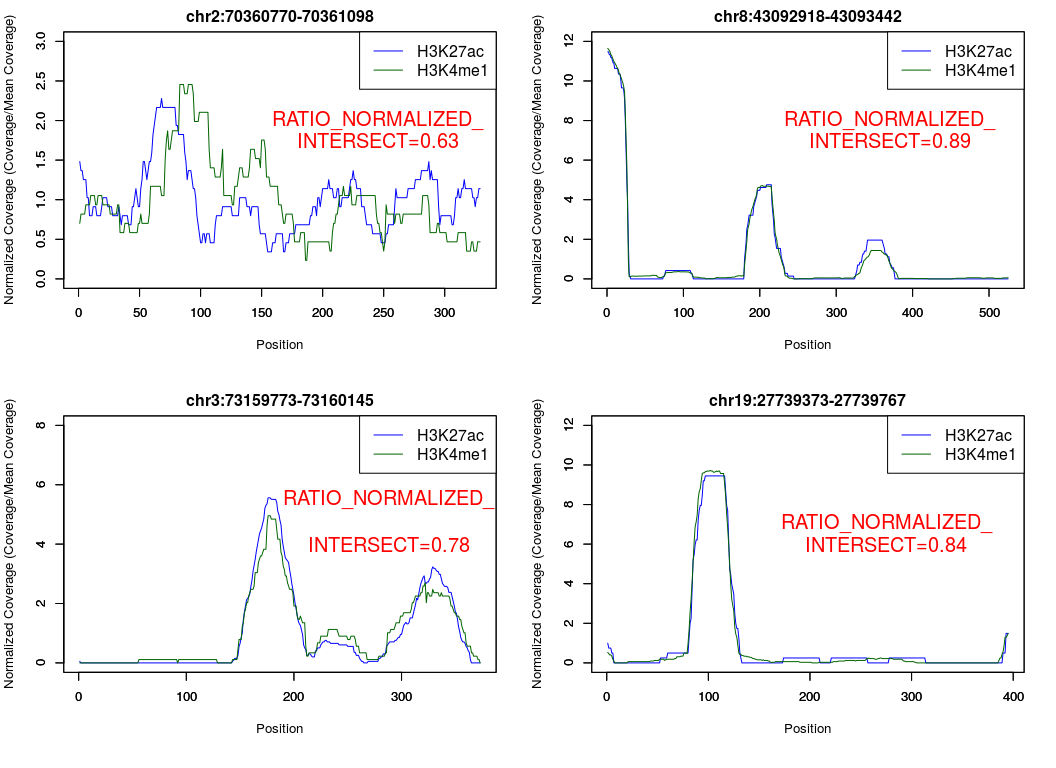metrics <- similarity(demoProfiles$chr2.70360770.70361098$H3K27ac,
demoProfiles$chr2.70360770.70361098$H3K4me1)
metrics$metric$RATIO_NORMALIZED_INTERSECT
##  0.6316846

metrics <- similarity(demoProfiles$chr8.43092918.43093442$H3K27ac,
demoProfiles$chr8.43092918.43093442$H3K4me1)
metrics$metric$RATIO_NORMALIZED_INTERSECT
##  0.8914731

metrics <- similarity(demoProfiles$chr3.73159773.73160145$H3K27ac,
demoProfiles$chr3.73159773.73160145$H3K4me1)
metrics$metric$RATIO_NORMALIZED_INTERSECT
##  0.7832524

metrics <- similarity(demoProfiles$chr19.27739373.27739767$H3K27ac,
demoProfiles$chr19.27739373.27739767$H3K4me1)
metrics$metric$RATIO_NORMALIZED_INTERSECT
##  0.8439258

#### 1.4.2.6 SPEARMAN_CORRELATION Metric

The SPEARMAN_CORRELATION metric is the Spearman’s rho statistic calculated usign the two profiles.NA if minimal standard deviation (spearmanCorrSDThreashold parameter) is not respected for at least one of the profiles.

The SPEARMAN_CORRELATION assesses how well the relationship between the two ChIP-Seq profiles can be described using a monotonic function. As the RATIO_NORMALIZED_INTERSECT metric, the SPEARMAN_CORRELATION metric can be useful to detect regions with possibily similar profiles even if their have different amplitude.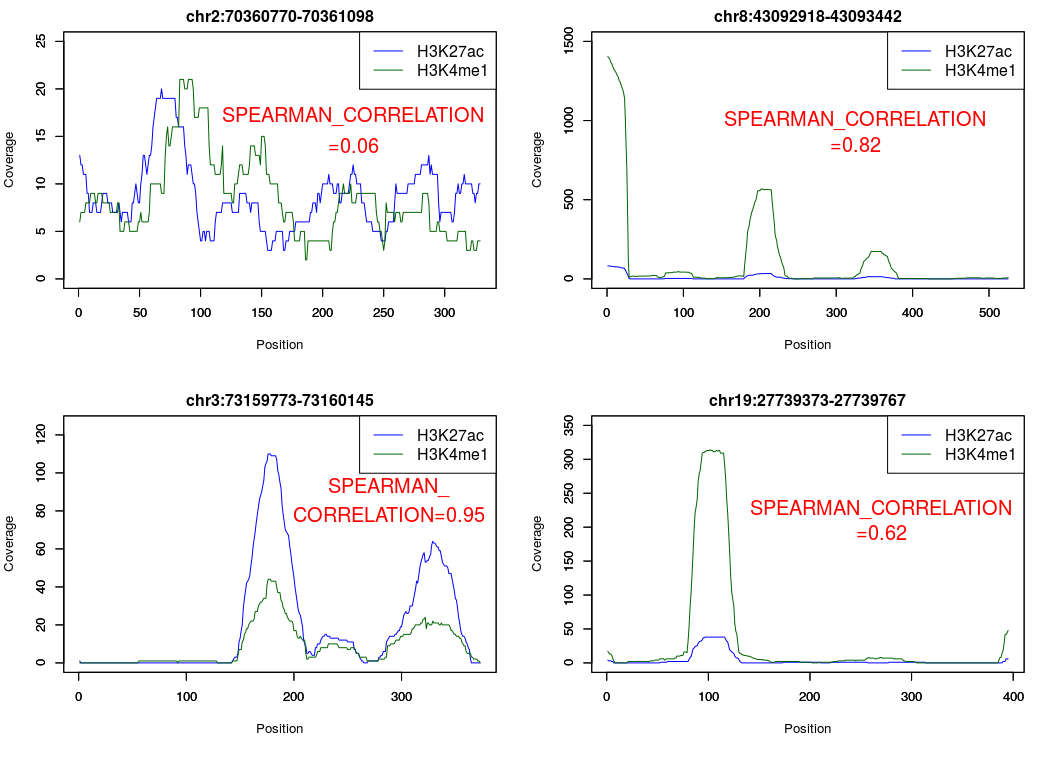metrics <- similarity(demoProfiles$chr2.70360770.70361098$H3K27ac,
demoProfiles$chr2.70360770.70361098$H3K4me1)
metrics$metric$SPEARMAN_CORRELATION
##  0.05945987

metrics <- similarity(demoProfiles$chr8.43092918.43093442$H3K27ac,
demoProfiles$chr8.43092918.43093442$H3K4me1)
metrics$metric$SPEARMAN_CORRELATION
##  0.819497

metrics <- similarity(demoProfiles$chr3.73159773.73160145$H3K27ac,
demoProfiles$chr3.73159773.73160145$H3K4me1)
metrics$metric$SPEARMAN_CORRELATION
##  0.9515006

metrics <- similarity(demoProfiles$chr19.27739373.27739767$H3K27ac,
demoProfiles$chr19.27739373.27739767$H3K4me1)
metrics$metric$SPEARMAN_CORRELATION
##  0.6164638

## 1.5 Using similaRpeak on real ChIP-Seq profiles

Highly active enhancer regions are thought to be important for the cell fate (Andersson et al. 2014, FANTOM5 consortium, Hnisz et al. 2013). Highly active enhancers regions have been selected in GM12878 cells. Similarity of ChIP-seq profiles has been tested using two histone post-transcriptional modifications linked to highly active enhancers H3K27ac (DCC accession: ENCFF000ASG) and H3K4me1 (DCC accession: ENCFF000ARY) from the Encyclopedia of DNA Elements (ENCODE) data (Dunham I et al. 2012). Accordingly with the literature, similarity between the profiles of these two histone marks has been identified.

First, the similaRpeak package must be loaded.

suppressMessages(library(similaRpeak))

A region, chr7:61968807-61969730, shows interesting profiles for both histones. Let’s load the data for this region.

data(chr7Profiles)
str(chr7Profiles)
## List of 1
##  $chr7.61968807.61969730:List of 2 ## ..$ H3K27ac: num [1:924] 31 31 31 31 30 29 27 24 24 26 ...
##   ..$H3K4me1: num [1:924] 319 317 310 300 284 274 259 257 258 253 ... H3K27ac and H3K4me1 profiles have those shapes: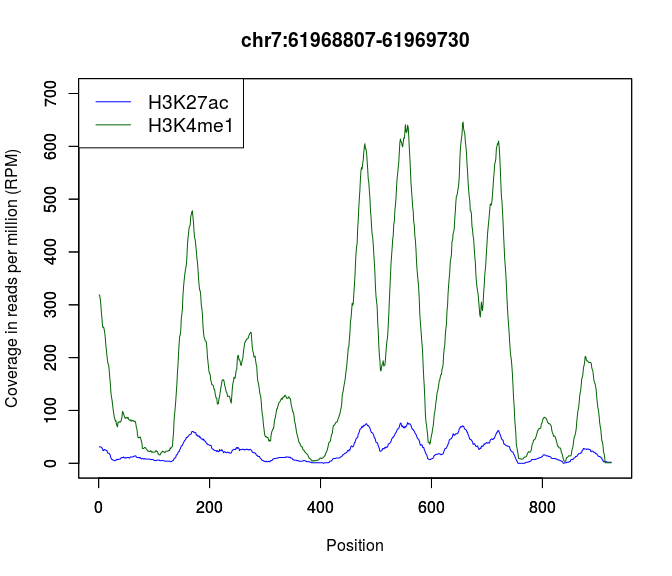The metrics are calculated using the similarity function which takes as arguments the two ChIP-Seq profiles vectors and the threshold values. metrics <- similarity(chr7Profiles$chr7.61968807.61969730$H3K27ac, chr7Profiles$chr7.61968807.61969730$H3K4me1, ratioAreaThreshold=5, ratioMaxMaxThreshold=2, ratioIntersectThreshold=5, ratioNormalizedIntersectThreshold=2, diffPosMaxThresholdMinValue=10, diffPosMaxThresholdMaxDiff=100, diffPosMaxTolerance=0.01) The similarity function returns a list which contains the general information about both ChIP-Seq profiles and a list of all calculated metrics. metrics ##$nbrPosition
##  924
##
## $areaProfile1 ##  22140 ## ##$areaProfile2
##  182991
##
## $maxProfile1 ##  77 ## ##$maxProfile2
##  646
##
## $maxPositionProfile1 ##  557 ## ##$maxPositionProfile2
##  657
##
## $metrics ##$metrics$RATIO_AREA ##  0.1209896 ## ##$metrics$DIFF_POS_MAX ##  -49.5 ## ##$metrics$RATIO_MAX_MAX ##  0.119195 ## ##$metrics$RATIO_INTERSECT ##  0.1208916 ## ##$metrics$RATIO_NORMALIZED_INTERSECT ##  0.8478406 ## ##$metrics$SPEARMAN_CORRELATION ##  0.9689683 Each specific information can be directly accessed. Some examples: metrics$areaProfile1
##  22140
metrics$areaProfile2 ##  182991 metrics$metrics$RATIO_INTERSECT ##  0.1208916 The RATIO_INTERSECT value of 0.12 and the RATIO_MAX_MAX value of 0.12 are quite low. Both values can be explained by the large difference in coverage between profiles. Those values could be interpreted as two profiles with low level of similarity. However, the RATIO_NORMALIZED_INTERSECT of 0.85 is much closer to 1. It could be a sign that the profiles, once normalized, are quite similar. This hypothesis can be validated by looking at a graph of the normalized profiles :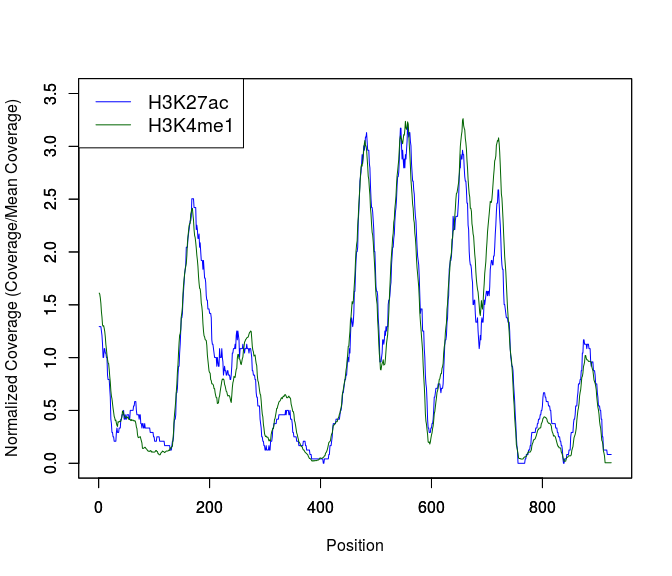## 1.6 Metrics calculation using a MetricFactory object It is possible to create only one selected metric by using the MetricFactory object (with the possibility of specifying the thresholds) and by passing the name of the metric to create (RATIO_AREA, DIFF_POS_MAX, RATIO_MAX_MAX, RATIO_INTERSECT or RATIO_NORMALIZED_INTERSECT): factory = MetricFactory$new(diffPosMaxTolerance=0.04)

The factory has to be iniatized only once and can be used as many times as necessary. It can calculate the same metrics but with different profiles or different metrics with same profiles as long as the thresholds values remain the same:

ratio_max_max <- factory$createMetric(metricType="RATIO_MAX_MAX", profile1=demoProfiles$chr2.70360770.70361098$H3K27ac, profile2=demoProfiles$chr2.70360770.70361098$H3K4me1) ratio_max_max ##$RATIO_MAX_MAX
##  0.952381

ratio_normalized_intersect <- factory$createMetric( metricType="RATIO_NORMALIZED_INTERSECT", profile1=demoProfiles$chr2.70360770.70361098$H3K27ac, profile2=demoProfiles$chr2.70360770.70361098$H3K4me1) ratio_normalized_intersect ##$RATIO_NORMALIZED_INTERSECT
##  0.6316846

## 1.7 Session info

Here is the output of sessionInfo() on the system on which this document was compiled:

## R version 3.3.1 (2016-06-21)
## Platform: x86_64-pc-linux-gnu (64-bit)
## Running under: Ubuntu 16.04.1 LTS
##
## locale:
##   LC_CTYPE=en_US.UTF-8       LC_NUMERIC=C
##   LC_TIME=en_US.UTF-8        LC_COLLATE=C
##   LC_MONETARY=en_US.UTF-8    LC_MESSAGES=en_US.UTF-8
##   LC_PAPER=en_US.UTF-8       LC_NAME=C
##  LC_MEASUREMENT=en_US.UTF-8 LC_IDENTIFICATION=C
##
## attached base packages:
##  stats4    parallel  stats     graphics  grDevices utils     datasets
##  methods   base
##
## other attached packages:
##   rtracklayer_1.34.0         GenomicAlignments_1.10.0
##   Rsamtools_1.26.0           Biostrings_2.42.0
##   XVector_0.14.0             SummarizedExperiment_1.4.0
##   Biobase_2.34.0             GenomicRanges_1.26.0
##   GenomeInfoDb_1.10.0        IRanges_2.8.0
##  S4Vectors_0.12.0           BiocGenerics_0.20.0
##  similaRpeak_1.6.0          R6_2.2.0
##  knitr_1.14                 BiocStyle_2.2.0
##
## loaded via a namespace (and not attached):
##   Rcpp_0.12.7        magrittr_1.5       zlibbioc_1.20.0
##   BiocParallel_1.8.0 lattice_0.20-34    stringr_1.1.0
##   tools_3.3.1        grid_3.3.1         htmltools_0.3.5
##  yaml_2.1.13        assertthat_0.1     digest_0.6.10
##  tibble_1.2         Matrix_1.2-7.1     formatR_1.4
##  bitops_1.0-6       RCurl_1.95-4.8     evaluate_0.10
##  rmarkdown_1.1      stringi_1.1.2      XML_3.98-1.4